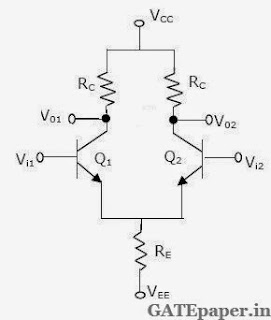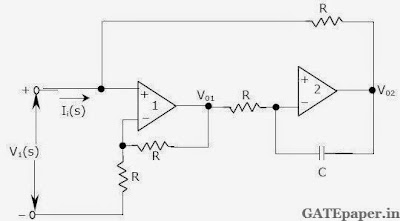### GATE 1996 ECE Analog Circuits (Analog Electronics) - Video Solutions

1. In the circuit of the given figure, assume that the diodes are ideal and the meter is an average indicating ammeter. The ammeter will read ________2. The circuit shown in the figure is that ofa. A Non-inverting amplifier
b. An Inverting amplifier
c. An Oscillator
d. A Schmitt trigger

3. In the circuit shown, ‘N’ is a finite gain amplifier with a gain of K, large input impedance and very low output impedance. The input impedance of the feedback amplifier with the feedback impedance Z connected as shown will be ______________4. A Darlington stage is shown in the figure. If the Transconductance of Q1 is gm1 and Q2 is gm2, then the overall Transconductance gmc ≡ [icc/vbec] is given bya. gm1
b. 0.5 gm1
c. gm2
d. 0.5 gm2

5. Value of R in the oscillator circuit shown in the given figure, so chosen that it just oscillates at an angular frequency of ω. The value of ω and the required value of R will respectively area. 105 rad/sec, 2 x 104
b. 2 x 104 rad/sec, 2 x 104
c. 2 x 104 rad/sec, 105

6. A common emitter amplifier with an external capacitors CC connected across the base and the collector of the transistor is shown. Given gm = 5 mA/V, rπ = 20 kΩ, Cπ = 1.5 pF and Cµ = 0.5 pF.a. Determine the ac small signal mid band voltage gain, Vo/Vs.
b. Determine the upper cutoff frequency fH of the amplifier.

7. A resistively loaded and resistively biased differential amplifier circuit is shown. Neglect base current and assume matched transistors with VA -> ∞ and β = 100. Use VT = 26 mV, VBE(on) = 0.7 volts and VCE(sat) = 0.1 volts.a. Determine the values of RC and RE to meet the following specifications: double ended differential mode gain = 500, CMRR = 500 and differential mode input resistance of 2 MΩ.
b. Determine the minimum values of VCC and VEE such that the transistors remain in the forward active region under zero signal condition. Assume that the DC common mode input is zero.

8. Assuming ideal operational amplifiers, show that the circuit shown simulates in inductor i.e. show that Vi(S)/Ii(S) is inductive and write the expression for the effective inductance.